# please solve #7. 6. A tension test specimen of a certain material has a diameter of...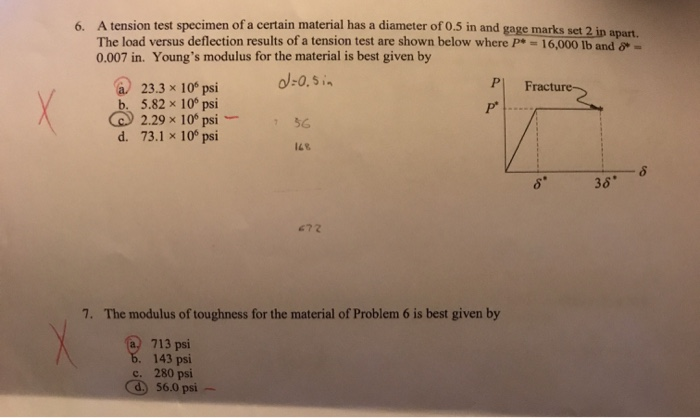6. A tension test specimen of a certain material has a diameter of 0.5 in and gage marks set 2 in The load versus deflection results of a tension test are shown below where p = 16,000 lb and 0.007 in. Young's modulus for the material is best given by a 23.3 x 10 psi d=0.5in b. 5.82 x 10 psi 2.29 x 10psi d. 73.1 x 10º psi P] Fractures 56 38 672 7. The modulus of toughness for the material of Problem 6 is best given by a, 713 psi b. 143 psi c. 280 psi d. 56.0 psi -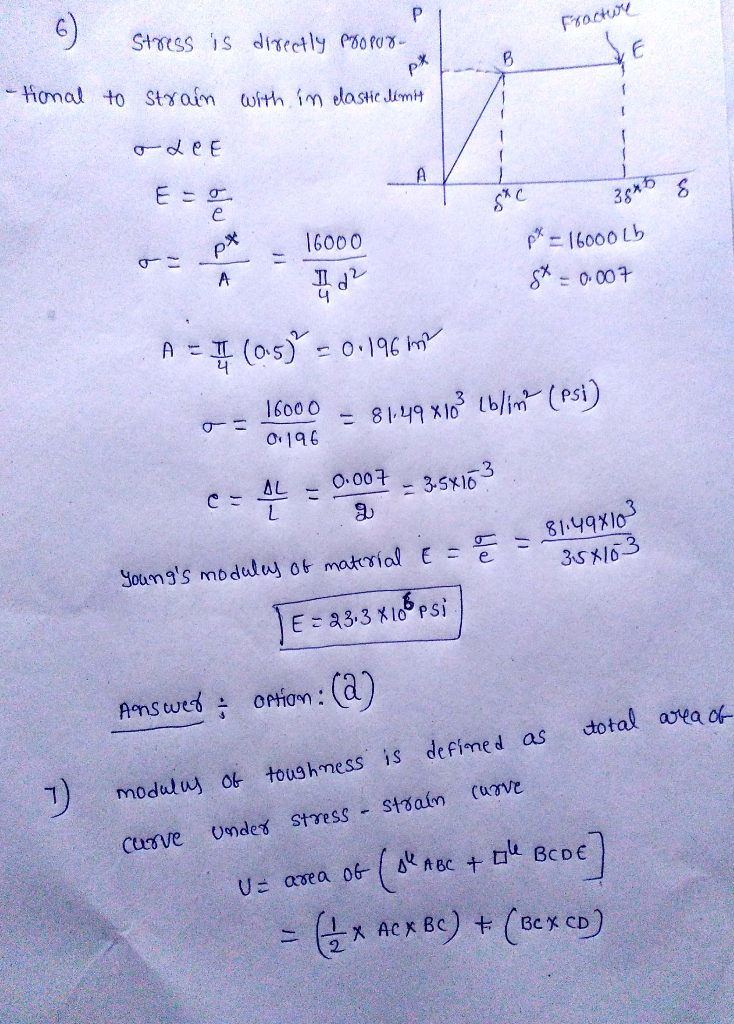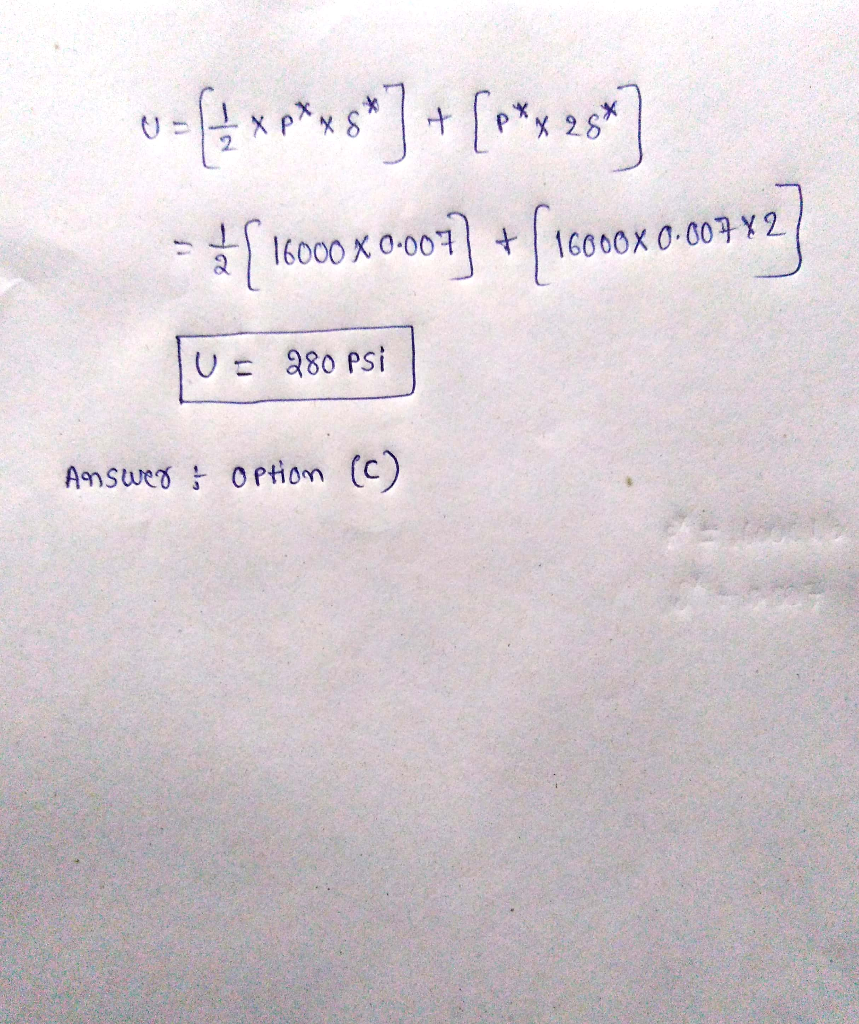#### Earn Coin

Coins can be redeemed for fabulous gifts.

Similar Homework Help Questions
• ### Problem 1: A tension test was performed on a steel specimen having an original diameter of...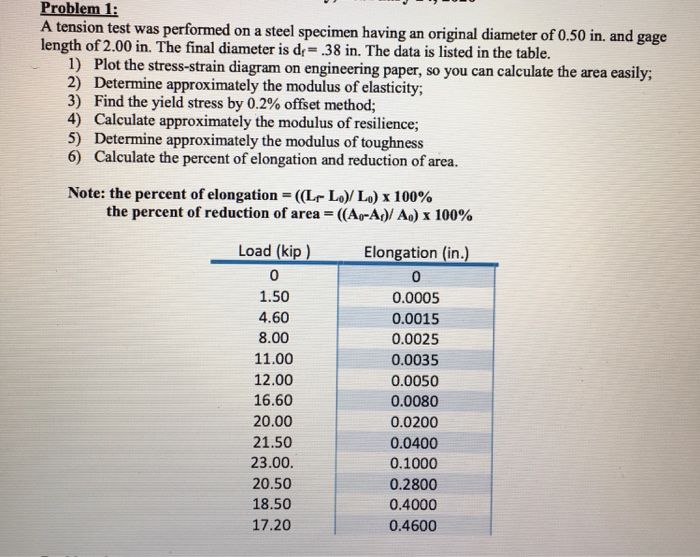Problem 1: A tension test was performed on a steel specimen having an original diameter of 0.50 in. and gage length of 2.00 in. The final diameter is de .38 in. The data is listed in the table. 1) Plot the stress-strain diagram on engineering paper, so you can calculate the area easily; 2) Determine approximately the modulus of elasticity; 3) Find the yield stress by 0.2% offset method; 4) Calculate approximately the modulus of resilience; 5) Determine approximately the...

• ### ire of initial length Sa is run over a frictionless pulley as shown. The weight W...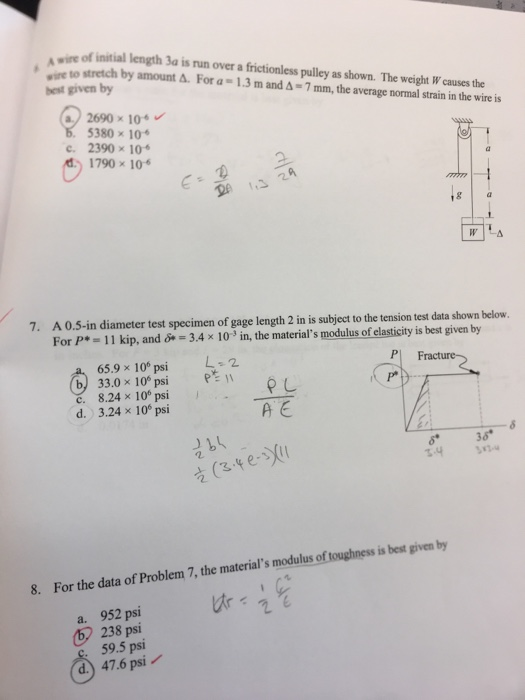ire of initial length Sa is run over a frictionless pulley as shown. The weight W causes the ire to stretch by amount A. For a- 1.3 m and A-7 mm, the average normal strain in the wire is best given by 2690 x 10 380 x 10 e. 2390 x 10 1790 x 10 DA 13 29 7. A 0.5-in diameter test specimen of gage length 2 in is subject to the tension test data shown below. For P*-11...

• ### A Grade 2 Titanium tension test specimen has a diameter of 12.60 mm and a gage...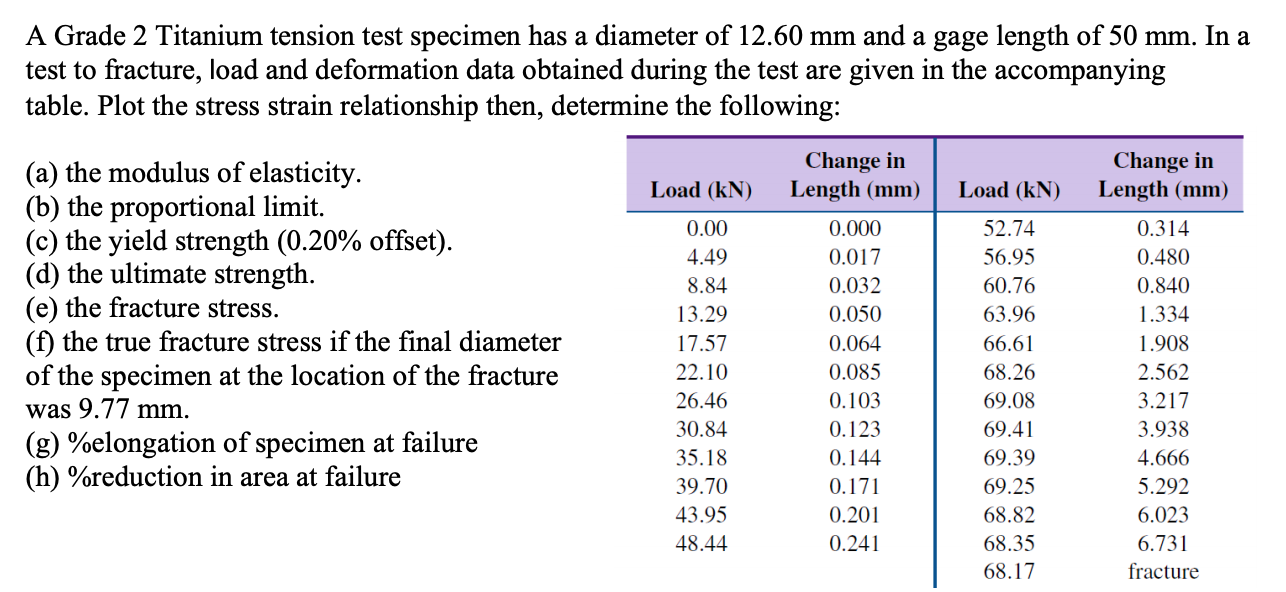A Grade 2 Titanium tension test specimen has a diameter of 12.60 mm and a gage length of 50 mm. In a test to fracture, load and deformation data obtained during the test are given in the accompanying table. Plot the stress strain relationship then, determine the following: (a) the modulus of elasticity. (b) the proportional limit. (c) the yield strength (0.20% offset). (d) the ultimate strength. (e) the fracture stress. (f) the true fracture stress if the final diameter...